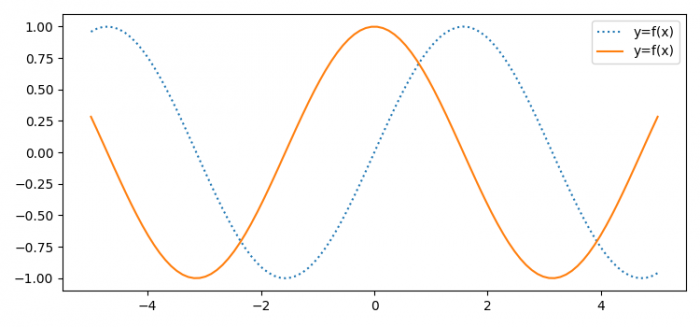# How to make two markers share the same label in the legend using Matplotlib?

To make two markers share the same label in the legend using Matplotlib, we can take the following steps

## Steps

• Set the figure size and adjust the padding between and around the subplots.
• Create x and y data points using numpy.
• Plot x and y(as a sin(x) and cos(x)), using plot() method.
• Place legend with location=1.
• To display the figure, use show() method.

## Example

import numpy as np
import matplotlib.pyplot as plt

plt.rcParams["figure.figsize"] = [7.50, 3.50]
plt.rcParams["figure.autolayout"] = True
x = np.linspace(-5, 5, 100)

plt.plot(x, np.sin(x), ls="dotted", label='y=f(x)')
plt.plot(x, np.cos(x), ls="-", label='y=f(x)')
plt.legend(loc=1)

plt.show()

## OutputIt is not recommended to make two markers share the same label in the legend because different plots should have different markers and labels.

Updated on: 03-Jun-2021

638 Views